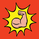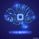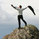28306 vizualizações
28306
This is version 2 of my ANN strategy. This version will not repaint, but requires some settings configuration.
The reason why the old version repainted, is because it used the daily OHLC/4 which kept changing, causing lower timeframes ro repaint.
You can now specify which timeframes to use. The bigger timeframe is set in the settings (e.g. 1 day). You set the smaller settings by opening a certain chart (e.g. a 4h chart).
The timeframes are used to calculate the percentual difference, which is fed to the ANN as input

Link to version 1:
//@version=2
strategy("ANN Strategy v2")

threshold = input(title="Threshold", type=float, defval=0.0000, step=0.0001)
timeframe = input(title="Timeframe", type=resolution, defval='1D' )

getDiff() =>
yesterday=security(tickerid, timeframe, ohlc4)
today=ohlc4
delta=today-yesterday
percentage=delta/yesterday

PineActivationFunctionLinear(v) => v
PineActivationFunctionTanh(v) => (exp(v) - exp(-v))/(exp(v) + exp(-v))

l0_0 = PineActivationFunctionLinear(getDiff())

l1_0 = PineActivationFunctionTanh(l0_0*0.8446488687)
l1_1 = PineActivationFunctionTanh(l0_0*-0.5674069006)
l1_2 = PineActivationFunctionTanh(l0_0*0.8676766445)
l1_3 = PineActivationFunctionTanh(l0_0*0.5200611473)
l1_4 = PineActivationFunctionTanh(l0_0*-0.2215499554)

l2_0 = PineActivationFunctionTanh(l1_0*0.3341657935 + l1_1*-2.0060003664 + l1_2*0.8606354375 + l1_3*0.9184846912 + l1_4*-0.8531172267)
l2_1 = PineActivationFunctionTanh(l1_0*-0.0394076437 + l1_1*-0.4720374911 + l1_2*0.2900968524 + l1_3*1.0653326022 + l1_4*0.3000188806)
l2_2 = PineActivationFunctionTanh(l1_0*-0.559307785 + l1_1*-0.9353655177 + l1_2*1.2133832962 + l1_3*0.1952686024 + l1_4*0.8552068166)
l2_3 = PineActivationFunctionTanh(l1_0*-0.4293220754 + l1_1*0.8484259409 + l1_2*-0.7154087313 + l1_3*0.1102971055 + l1_4*0.2279392724)
l2_4 = PineActivationFunctionTanh(l1_0*0.9111779155 + l1_1*0.2801691115 + l1_2*0.0039982713 + l1_3*-0.5648257117 + l1_4*0.3281705155)
l2_5 = PineActivationFunctionTanh(l1_0*-0.2963954503 + l1_1*0.4046532178 + l1_2*0.2460580977 + l1_3*0.6608675819 + l1_4*-0.8732022547)
l2_6 = PineActivationFunctionTanh(l1_0*0.8810811932 + l1_1*0.6903706878 + l1_2*-0.5953059103 + l1_3*-0.3084040686 + l1_4*-0.4038498853)
l2_7 = PineActivationFunctionTanh(l1_0*-0.5687101164 + l1_1*0.2736758588 + l1_2*-0.2217360382 + l1_3*0.8742950972 + l1_4*0.2997583987)
l2_8 = PineActivationFunctionTanh(l1_0*0.0708459913 + l1_1*0.8221730616 + l1_2*-0.7213265567 + l1_3*-0.3810462836 + l1_4*0.0503867753)
l2_9 = PineActivationFunctionTanh(l1_0*0.4880140595 + l1_1*0.9466627196 + l1_2*1.0163097961 + l1_3*-0.9500386514 + l1_4*-0.6341709382)
l2_10 = PineActivationFunctionTanh(l1_0*1.3402207103 + l1_1*0.0013395288 + l1_2*3.4813009133 + l1_3*-0.8636814677 + l1_4*41.3171047132)
l2_11 = PineActivationFunctionTanh(l1_0*1.2388217292 + l1_1*-0.6520886912 + l1_2*0.3508321737 + l1_3*0.6640560714 + l1_4*1.5936220597)
l2_12 = PineActivationFunctionTanh(l1_0*-0.1800525171 + l1_1*-0.2620989752 + l1_2*0.056675277 + l1_3*-0.5045395315 + l1_4*0.2732553554)
l2_13 = PineActivationFunctionTanh(l1_0*-0.7776331454 + l1_1*0.1895231137 + l1_2*0.5384918862 + l1_3*0.093711904 + l1_4*-0.3725627758)
l2_14 = PineActivationFunctionTanh(l1_0*-0.3181583022 + l1_1*0.2467979854 + l1_2*0.4341718676 + l1_3*-0.7277619935 + l1_4*0.1799381758)
l2_15 = PineActivationFunctionTanh(l1_0*-0.5558227731 + l1_1*0.3666152536 + l1_2*0.1538243225 + l1_3*-0.8915928174 + l1_4*-0.7659355684)
l2_16 = PineActivationFunctionTanh(l1_0*0.6111516061 + l1_1*-0.5459495224 + l1_2*-0.5724238425 + l1_3*-0.8553500765 + l1_4*-0.8696190472)
l2_17 = PineActivationFunctionTanh(l1_0*0.6843667454 + l1_1*0.408652181 + l1_2*-0.8830470112 + l1_3*-0.8602324935 + l1_4*0.1135462621)
l2_18 = PineActivationFunctionTanh(l1_0*-0.1569048216 + l1_1*-1.4643247888 + l1_2*0.5557152813 + l1_3*1.0482791924 + l1_4*1.4523116833)
l2_19 = PineActivationFunctionTanh(l1_0*0.5207514017 + l1_1*-0.2734444192 + l1_2*-0.3328660936 + l1_3*-0.7941515963 + l1_4*-0.3536051491)
l2_20 = PineActivationFunctionTanh(l1_0*-0.4097807954 + l1_1*0.3198619826 + l1_2*0.461681627 + l1_3*-0.1135575498 + l1_4*0.7103339851)
l2_21 = PineActivationFunctionTanh(l1_0*-0.8725014237 + l1_1*-1.0312091401 + l1_2*0.2267643037 + l1_3*-0.6814258121 + l1_4*0.7524828703)
l2_22 = PineActivationFunctionTanh(l1_0*-0.3986855003 + l1_1*0.4962556631 + l1_2*-0.7330224516 + l1_3*0.7355772164 + l1_4*0.3180141739)
l2_23 = PineActivationFunctionTanh(l1_0*-1.083080442 + l1_1*1.8752543187 + l1_2*0.3623326265 + l1_3*-0.348145191 + l1_4*0.1977935038)
l2_24 = PineActivationFunctionTanh(l1_0*-0.0291290625 + l1_1*0.0612906199 + l1_2*0.1219696687 + l1_3*-1.0273685429 + l1_4*0.0872219768)
l2_25 = PineActivationFunctionTanh(l1_0*0.931791094 + l1_1*-0.313753684 + l1_2*-0.3028724837 + l1_3*0.7387076712 + l1_4*0.3806140391)
l2_26 = PineActivationFunctionTanh(l1_0*0.2630619402 + l1_1*-1.9827996702 + l1_2*-0.7741413496 + l1_3*0.1262957444 + l1_4*0.2248777886)
l2_27 = PineActivationFunctionTanh(l1_0*-0.2666322362 + l1_1*-1.124654664 + l1_2*0.7288282621 + l1_3*-0.1384289204 + l1_4*0.2395966188)
l2_28 = PineActivationFunctionTanh(l1_0*0.6611845175 + l1_1*0.0466048937 + l1_2*-0.1980999993 + l1_3*0.8152350927 + l1_4*0.0032723211)
l2_29 = PineActivationFunctionTanh(l1_0*-0.3150344751 + l1_1*0.1391754608 + l1_2*0.5462816249 + l1_3*-0.7952302364 + l1_4*-0.7520712378)
l2_30 = PineActivationFunctionTanh(l1_0*-0.0576916066 + l1_1*0.3678415302 + l1_2*0.6802537378 + l1_3*1.1437036331 + l1_4*-0.8637405666)
l2_31 = PineActivationFunctionTanh(l1_0*0.7016273068 + l1_1*0.3978601709 + l1_2*0.3157049654 + l1_3*-0.2528455662 + l1_4*-0.8614146703)
l2_32 = PineActivationFunctionTanh(l1_0*1.1741126834 + l1_1*-1.4046408959 + l1_2*1.2914477803 + l1_3*0.9904052964 + l1_4*-0.6980155826)

l3_0 = PineActivationFunctionTanh(l2_0*-0.1366382003 + l2_1*0.8161960822 + l2_2*-0.9458773183 + l2_3*0.4692969576 + l2_4*0.0126710629 + l2_5*-0.0403001012 + l2_6*-0.0116244898 + l2_7*-0.4874816289 + l2_8*-0.6392241448 + l2_9*-0.410338398 + l2_10*-0.1181027081 + l2_11*0.1075562037 + l2_12*-0.5948728252 + l2_13*0.5593677345 + l2_14*-0.3642935247 + l2_15*-0.2867603217 + l2_16*0.142250271 + l2_17*-0.0535698019 + l2_18*-0.034007685 + l2_19*-0.3594532426 + l2_20*0.2551095195 + l2_21*0.4214344983 + l2_22*0.8941621336 + l2_23*0.6283377368 + l2_24*-0.7138020667 + l2_25*-0.1426738249 + l2_26*0.172671223 + l2_27*0.0714824385 + l2_28*-0.3268182144 + l2_29*-0.0078989755 + l2_30*-0.2032828145 + l2_31*-0.0260631534 + l2_32*0.4918037012)

buying = l3_0 > 0 ? true : l3_0 < -0 ? false : buying

hline(0, title="base line")
//bgcolor(l3_0 > 0.0014 ? green : l3_0 < -0.0014 ? red : gray, transp=20)
bgcolor(buying ? green : red, transp=20)
plot(l3_0, color=silver, style=area, transp=75)
plot(l3_0, color=aqua, title="prediction")

if (longCondition)
strategy.entry("Long", strategy.long)

shortCondition = buying != true
if (shortCondition)
strategy.entry("Short", strategy.short)Hello, have been trying to add a date range condition to the code so the "3000 order's limit" doesn't pop and block the backtesting in certain symbols, since picking the ideal daily chart timeframe takes as default decades of date (which I find mostly useless), I would like to shorten such date range to 5-6 years with this code.

##
from_day = input(defval = 1, title = "From Day", minval = 1)
from_month = input(defval = 3, title = "From Month", minval = 1)
from_year = input(defval = 2018, title = "From Year", minval = 1970)

to_day = input(defval = 1, title = "To Day", minval = 1)
to_month = input(defval = 1, title = "To Month", minval = 1)
to_year = input(defval = 2100, title = "To Year", minval = 1970)

time_cond = (time > timestamp(from_year, from_month, from_day, 00, 00)) and (time < timestamp(to_year, to_month, to_day, 23, 59))
##

Adding time_cond to the strategy_entry, but I am unable to make it work, do you have any solution or version including a data range?
Resposta@sirolf2009 hi
What you and many who called using strategy for today's ohlc4 called cheating don't understand is a very simple fact that makes your version 2 much worse than version 1 !!!

let me show that with a simple picture

The top chart is the daily chart and the one bellow is 30 min chart, now, the candle that i marked on the bottom chart is the last candle of that day ! take a look, where would be the OHLC4 price on that 30 min candle? compare it to what you would see on the daily chart !!!!

There is a 200\$ GAP in this situation !!!!

No matter what you would use for current candle's value calculation, in pine script, there will ALWAYS be repainting issue, that is a bad programming on the base structure of trading view ( they can deny it as much as they like ! ). But when you choose to call the current value on current candle, you can introduce HUGE error margin into the code just by doing it !!!!
RespostaThis strategy does not work on the realtime
it has repaint ,
I would advise you to test my osilator (powerful bay signal) .
Resposta